Blog > Authors > Edsko de Vries

# An in-depth look at quickcheck-state-machine

28 January 2019 Edsko de Vries 46 mins readPlease note: this post originally appeared on the Well-Typed blog. Stateful APIs are everywhere: file systems, databases, widget libraries, the list goes on. Automated testing of such APIs requires generating sequences of API calls, and when we find a failing test, ideally shrinking such a sequence to a minimal test case. Neither the generation nor the shrinking of such sequences is trivial. After all, it is the very nature of stateful systems that later calls may depend on earlier calls: we can only add rows to a database table after we create it, we can only write to a file after we open it, etc. Such dependencies need to be tracked carefully. Moreover, in order to verify the responses we get back from the system, the test needs to maintain some kind of internal representation of what it thinks the internal state of the system is: when we read from a file, we need to know what was in the file in order to be able to verify if the response was correct or not.

In this blog post we will take an in-depth look at `quickcheck-state-machine`, a library for testing stateful code. Our running example will be the development of a simple mock file system that should behave identically to a real file system. Although simple, the example will be large enough to give us an opportunity to discuss how we can verify that our generator is producing all test cases we need, and how we can inspect whether the shrinker is doing a good job; in both cases, test case labelling will turn out to be essential. Throughout we will also discuss design patterns for quickcheck-state-machine tests which improve separation of concerns and reduce duplication. It should probably be pointed out that this is an opinionated piece: there are other ways to set things up than we present here.

We will not show the full development in this blog post, and instead focus on explaining the underlying concepts. If you want to follow along, the code is available for download. We will assume version 0.6 of quickcheck-state-machine, which was recently released. If you are using an older version, it is recommended to upgrade, since the newer version includes some important bug fixes, especially in the shrinker.

## Introducing the running example

Our running example will be the development of a simple mock file system; the intention is that its behaviour is identical to the real file system, within the confines of what it needs to support. We will represent the state of the file system as

``````data Mock = M {
dirs  :: Set Dir
, files :: Map File String
, open  :: Map MHandle File
, next  :: MHandle
}

type MHandle = Int

emptyMock :: Mock
emptyMock = M (Set.singleton (Dir [])) Map.empty Map.empty 0``````

We record which directories (folders) exist, the contents of all files on the system, the currently open handles (where mock handles are just integers), and the next available mock handle. To avoid confusion between files and directories we do not use FilePath but instead use

``````data Dir  = Dir [String]
data File = File {dir :: Dir, name :: String}``````

As one example, here is the mock equivalent of readFile:

``````type MockOp a = Mock -> (Either Err a, Mock)

mRead :: File -> MockOp String
mRead f m@(M _ fs hs _)
| alreadyOpen               = (Left Busy         , m)
| Just s <- Map.lookup f fs = (Right s           , m)
| otherwise                 = (Left DoesNotExist , m)
where
alreadyOpen = f `List.elem` Map.elems hs``````

We first check if there is an open handle to the file; if so, we disallow reading this file (“resource busy”); if the file exists, we return its content; otherwise we report a “does not exist” error. The implementation of the other mock functions is similar; the full API is

``````mMkDir :: Dir               -> MockOp ()
mOpen  :: File              -> MockOp MHandle
mWrite :: MHandle -> String -> MockOp ()
mClose :: MHandle           -> MockOp ()
mRead  :: File              -> MockOp String``````

Finally, we should briefly talk about errors; the errors that the mock file system can report are given by

``data Err = AlreadyExists | DoesNotExist | HandleClosed | Busy``

and they capture a subset of the IO exceptions1

``````fromIOError :: IOError -> Maybe Err
fromIOError e =
case ioeGetErrorType e of
GHC.NoSuchThing      -> Just DoesNotExist
GHC.ResourceBusy     -> Just Busy
GHC.IllegalOperation -> Just HandleClosed
_otherwise           -> Nothing``````

## Testing

Typically we are developing some stateful code, and then write a pure (mock) implementation of the same thing to test it, making sure that the stateful implementation and the simpler pure model compute the same things. Here we are doing the opposite: we are adjusting the model (the mock file system) to match what the real file system does. Either way, the process is the same: we write tests, execute them both in the real thing and in the model, and compare results.

If we were writing unit tests, we might write tests such as

• Write to two different files
• Write to a file and then read it
• etc.

However, as John Hughes of QuviQ – and one of the original authors of QuickCheck – likes to point out, as systems grow, it becomes impossible to write unit tests that test the interaction between all the features of the system. So, don’t write unit tests. Instead, generate tests, and verify properties.

To generate tests for our mock file system, we have to generate sequences of calls into the API; “open this file, open that file, write to the first file we opened, …”. We then execute this sequence both against the mock file system and against the real thing, and compare results. If something goes wrong, we end up with a test case that we can inspect. Ideally, we should then try to reduce this test to try and construct a minimal test case that illustrates the bug. We have to be careful when shrinking: for example, when we remove a call to open from a test case, then any subsequent writes that used that file handle must also be removed. A library such as `quickcheck-state-machine` can be used both to help with generating such sequences and, importantly, with shrinking them.

## Reifying the API

It is important that we generate the test before executing it. In other words, the test generation should not depend on any values that we only get when we run the test. Such a dependency makes it impossible to re-run the same test multiple times (no reproducible test cases) or shrink tests to obtain minimal examples. In order to do this, we need to reify the API: we need to define a data type whose constructors correspond to the API calls:

``````data Cmd h =
MkDir Dir
| Open File
| Write h String
| Close h

`Cmd` is polymorphic in the type of handles h; this is important, because we should be able to execute commands both against the mock file system and against the real file system:

``````runMock ::       Cmd MHandle -> Mock -> (.. Mock)
runIO   :: .. -> Cmd IO.Handle -> IO ..``````

What should the return type of these functions be? After all, different functions return different things: Open returns a new handle, Read returns a string, the other functions return unit. To solve this problem we will simply introduce a union type2 for successful responses

``data Success h = Unit () | Handle h | String String``

A response is then either a succesful response or an error:

``newtype Resp h = Resp (Either Err (Success h))``

It is now easy to implement runMock: we just map all the constructors in Cmd to the corresponding API calls, and wrap the result in the appropriate constructor of Success:

``````runMock :: Cmd MHandle -> Mock -> (Resp MHandle, Mock)
runMock (MkDir d)   = first (Resp . fmap Unit)   . mMkDir d
runMock (Open  f)   = first (Resp . fmap Handle) . mOpen  f
runMock (Write h s) = first (Resp . fmap Unit)   . mWrite h s
runMock (Close h)   = first (Resp . fmap Unit)   . mClose h
runMock (Read  f)   = first (Resp . fmap String) . mRead  f``````

where `first :: (a -> b) -> (a, x) -> (b, x)` comes from `Data.Bifunctor`.

We can write a similar interpreter for IO; it will take a FilePath as an additional argument that it will use as a prefix for all paths; we will use this to run the IO test in some temporary directory.

``runIO :: FilePath -> Cmd IO.Handle -> IO (Resp IO.Handle)``

## References

Our interpreter for IO takes real IO handles as argument; but will not have any real handles until we actually run the test. We need a way to generate commands that run in IO but don’t use real handles (yet). Here is where we see the first bit of infrastructure provided by `quickcheck-state-machine`, references:

``data Reference a r = Reference (r a)``

where we will instantiate that `r` parameter to either `Symbolic` or `Concrete`:3

``````data Symbolic a = Symbolic Var
data Concrete a = Concrete a``````

In other words, a `Reference a Concrete` is really just a wrapper around an `a`; indeed, `quickcheck-state-machine` provides

``````reference :: a -> Reference a Concrete
concrete  :: Reference a Concrete -> a``````

However, a `Reference a Symbolic` is a variable:

``newtype Var = Var Int``

An example of a program using symbolic references is

``````openThenWrite :: [Cmd (Reference IO.Handle Symbolic)]
openThenWrite = [
Open (File (Dir []) "a")
, Open (File (Dir []) "b")
, Write (Reference (Symbolic (Var 0))) "Hi"
]``````

This program corresponds precisely to our example from earlier: “open this file, open that file, then write to the first file we opened”. Commands can return as many symbolic references in their result values as they want4; in our simple example, only `Open` creates a new reference, and so `Var 0` returns to the handle returned by the first call to `Open`.

When we execute the test, those variables will be instantiated to their real values, turning symbolic references into concrete references. We will of course not write programs with symbolic references in them by hand; as we will see later, `quickcheck-state-machine` provides infrastructure for doing so.

Since we will frequently need to instantiate `Cmd` and `Resp` with references to handles, we will introduce some special syntax for this:

``````newtype At f r = At (f (Reference IO.Handle r))
type    f :@ r = At f r``````

For example, here is a wrapper around `runIO` that we will need that executes a command with concrete references:

``````semantics :: FilePath -> Cmd :@ Concrete -> IO (Resp :@ Concrete)
semantics root (At c) = (At . fmap reference) <\$>
runIO root (concrete <\$> c)``````

This is really just a call to `runIO`, with some type wrapping and unwrapping.

## Relating the two implementations

When we run our tests, we will execute the same set of commands against the mock implementation and in real IO, and compare the responses we get after each command. In order to compare, say, a command “write to this `MHandle`” against the mock file system to a command “write to this `IOHandle`” in IO, we need to know the relation between the mock handles and the IO handles. As it turns out, the most convenient way to store this mapping is as a mapping from references to the IO handles (either concrete or symbolic) to the corresponding mock handles.

``````type HandleRefs r = [(Reference IO.Handle r, MHandle)]

(!) :: Eq k => [(k, a)] -> k -> a
env ! r = fromJust (lookup r env)``````

Then to compare the responses from the mock file system to the responses from IO we need to keep track of the state of the mock file system and this mapping; we will refer to this as the model for our test:

``````data Model r = Model Mock (HandleRefs r)

initModel :: Model r
initModel = Model emptyMock []``````

The model must be polymorphic in `r`: during test generation we will instantiate `r` to `Symbolic`, and during test execution we will instantiate `r` to `Concrete`.

## Stepping the model

We want to work towards a function

``transition :: Eq1 r => Model r -> Cmd :@ r -> Resp :@ r -> Model r``

to step the model; we will gradually build up towards this. First, we can use the model to translate from commands or responses in terms of references to the corresponding commands or responses against the mock file system:

``````toMock :: (Functor f, Eq1 r) => Model r -> f :@ r -> f MHandle
toMock (Model _ hs) (At fr) = (hs !) <\$> fr``````

Specifically, this can be instantiated to

``toMock :: Eq1 r => Model r -> Cmd :@ r -> Cmd MHandle``

which means that if we have a command in terms of references, we can translate that command to the corresponding command for the mock file system and execute it:

``````step :: Eq1 r => Model r -> Cmd :@ r -> (Resp MHandle, Mock)
step m@(Model mock _) c = runMock (toMock m c) mock``````

In order to construct the full new model however we also need to know how to extend the handle mapping. We can compute this by comparing the response we get from the “real” semantics (`Resp :@ r`) to the response we get from the mock semantics (from `step`), and simply zip the handles from both responses together to obtain the new mapping. We wrap all this up into an event:

``````data Event r = Event {
before   :: Model  r
, cmd      :: Cmd :@ r
, after    :: Model  r
, mockResp :: Resp MHandle
}``````

and we construct an event from a model, the command we executed, and the response we got from the real implementation:

``````lockstep :: Eq1 r
=> Model   r
-> Cmd  :@ r
-> Resp :@ r
-> Event   r
lockstep m@(Model _ hs) c (At resp) = Event {
before   = m
, cmd      = c
, after    = Model mock' (hs <> hs')
, mockResp = resp'
}
where
(resp', mock') = step m c
hs' = zip (toList resp) (toList resp')``````

The function we mentioned at the start of this section is now easily derived:

``````transition :: Eq1 r => Model r -> Cmd :@ r -> Resp :@ r -> Model r
transition m c = after . lockstep m c``````

as well as a function that compares the mock response and the response from the real file system and checks that they are the same:

``````postcondition :: Model   Concrete
-> Cmd  :@ Concrete
-> Resp :@ Concrete
-> Logic
postcondition m c r = toMock (after e) r .== mockResp e
where
e = lockstep m c r``````

We will pass this function to `quickcheck-state-machine` to be run after every command it executes to make sure that the model and the real system do indeed return the same responses; it therefore does not need to be polymorphic in `r`. (`Logic` is a type introduced by `quickcheck-state-machine`; think of it as a boolean with some additional information, somewhat similar to `QuickCheck’s Property` type.)

Events will also be very useful when we label our tests; more on that later.

## Constructing symbolic responses

We mentioned above that we will not write programs with symbolic references in it by hand. Instead what will happen is that we execute commands in the mock file system, and then replace any of the generated handles by new variables. Most of this happens behind the scenes by `quickcheck-state-machine`, but we do need to give it this function to construct symbolic responses:

``````symbolicResp :: Model Symbolic
-> Cmd :@ Symbolic
-> GenSym (Resp :@ Symbolic)
symbolicResp m c = At <\$> traverse (const genSym) resp
where
(resp, _mock') = step m c``````

This function does what we just described: we use step to execute the command in the mock model, and then traverse the response, constructing a new (fresh) variable for each handle. `GenSym` is a monad defined in `quickcheck-state-machine` for the sole purpose of generating variables; we won’t use it anywhere else except in this function.

## Generating commands

To generate commands, `quickcheck-state-machine` requires a function that produces the next command given the current model; this function will be a standard `QuickCheck` generator. For our running example, the generator is easy to write:

``````generator :: Model Symbolic -> Maybe (Gen (Cmd :@ Symbolic))
generator (Model _ hs) = Just \$ QC.oneof \$ concat [
withoutHandle
, if null hs then [] else withHandle
]
where
withoutHandle :: [Gen (Cmd :@ Symbolic)]
withoutHandle = [
fmap At \$ MkDir <\$> genDir
, fmap At \$ Open  <\$> genFile
, fmap At \$ Read  <\$> genFile
]

withHandle :: [Gen (Cmd :@ Symbolic)]
withHandle = [
fmap At \$ Write <\$> genHandle <*> genString
, fmap At \$ Close <\$> genHandle
]

genDir :: Gen Dir
genDir = do
n <- QC.choose (0, 3)
Dir <\$> replicateM n (QC.elements ["x", "y", "z"])

genFile :: Gen File
genFile = File <\$> genDir <*> QC.elements ["a", "b", "c"]

genString :: Gen String
genString = QC.sized \$ \n -> replicateM n (QC.elements "ABC")

genHandle :: Gen (Reference IO.Handle Symbolic)
genHandle = QC.elements (map fst hs)``````

A few comments on the generator:

• When we generate paths, we choose from a very small set of directory and file names. We are not really interested in testing that, for example, our implementation is Unicode-safe here; by picking from a small known set we generate tests that are more likely to contain multiple references to the same file, without having to add special provisions to do so.
• We cannot, of course, generate handles out of nowhere; fortunately, the model tells us which handles we have available, and so `genHandle` just picks one at random from that. We do not limit the generator to picking open handles: it is important to also test the behaviour of the system in the error case where a program attempts to write to a closed handle.
• The elements function from `QuickCheck`, which picks a random element from a list, is partial: it will throw an error if the list is empty. We must be careful not to generate any commands requiring handles when we have no handles yet.
• The reason for the `Maybe` in the type signature is that in some circumstances a generator might be unable to generate any commands at all given a particular model state. We don’t need to take advantage of this feature and therefore always return Just a generator.

We can also define a shrinker for commands, but we can start with a shrinker that says that individual commands cannot be shrunk:

``````shrinker :: Model Symbolic -> Cmd :@ Symbolic -> [Cmd :@ Symbolic]
shrinker _ _ = []``````

## Putting it all together

In order to actually start generating and running tests, quickcheck-state-machine wants us to bundle all of this functionality up in a single record:

``````sm :: FilePath -> StateMachine Model (At Cmd) IO (At Resp)
sm root = StateMachine {
initModel     = initModel
, transition    = transition
, precondition  = precondition
, postcondition = postcondition
, invariant     = Nothing
, generator     = generator
, distribution  = Nothing
, shrinker      = shrinker
, semantics     = semantics root
, mock          = symbolicResp
}``````

We have defined all of these functions above, with the exception of `precondition`. When `quickcheck-state-machine` finds a failing test, it will try to shrink it to produce a smaller test case. It does this by trying to remove commands from the program and then checking if the resulting program still “makes sense”: does the precondition of all commands still hold?

The precondition should be as liberal as possible; for instance, the precondition should not require that a file handle is open before writing to it, because we should also check that errors are handled correctly. Typically, the only thing the precondition should verify is that commands do not refer to non-existent handles (i.e., if we remove a call to open, then subsequent uses of the handle returned by that call to open simply cannot be executed anymore). Thus, we will define:

``````precondition :: Model Symbolic -> Cmd :@ Symbolic -> Logic
precondition (Model _ hs) (At c) =
forall (toList c) (`elem` map fst hs)``````

Aside. Although we do not need it for our running example, one other thing a precondition might do is rule out test cases that we explicitly don’t want to test. For example, if our mock file system throws an error in some cases because a particular combination of arguments to a call is simply not supported, then we don’t want to flag this as a bug. A clean way to implement this is to extend the error type with a field that marks an error as an intentional limitation; then the precondition can simply rule out any commands that (in the model) would return an error with this mark. This keeps the definition of the precondition itself simple, and the logic of what is and what isn’t an intentional limitation lives in the implementation of the model itself.

## Running the tests

Apart from some additional type class instances (see the code), we are now ready to define and execute the actual test:

``````prop_sequential :: FilePath -> QC.Property
prop_sequential tmpDir =
forAllCommands (sm rootUnused) Nothing \$ \cmds ->
tstTmpDir <- liftIO \$ createTempDirectory tmpDir "QSM"
let sm' = sm tstTmpDir
(hist, _model, res) <- runCommands sm' cmds
prettyCommands sm' hist
\$ checkCommandNames cmds
\$ res QC.=== Ok

rootUnused :: FilePath
rootUnused = error "root not used during command generation"``````

All functions prefixed `QC` are standard `QuickCheck` functions. The others come from `quickcheck-state-machine`:

• `forAllCommands` uses `QuickCheck`’s `forAllShrink` and instantiates it with the command generation and shrinking infrastructure from `quickcheck-state-machine`.
• `runCommands` then executes the generated commands, validating the postcondition at every step.
• `prettyCommands` renders those commands in case of a test failure.
• `checkCommandNames` adds some statistics about the distribution of commands in the generated tests.

We can run the test in `ghci`:

``````> quickCheck (prop_sequential "./tmp")
+++ OK, passed 100 tests:
56% coverage

3% []
2% [("MkDir",1)]
...``````

It tells us that all tests passed, and gives us some statistics about the tests that were executed: in 3% of the cases, they contained a single `MkDir` and a single `Read`, 3% were completely empty, 2% contained one call to `MkDir`, one call to `Open`, one call to `Read`, and so on.

## Labelling

At this point you might conclude that you’re done. We have the real implementation, we have the mock implementation, they return identical results for all tests, what else could we want?

Let’s think back to those unit tests we were on the verge of writing, but stopped just in time because we remembered that we should generate unit tests, not write them:

• Write to two different files
• Write to a file and then read it

How do we know that our generated tests include these two cases (and all the other unit tests that we would have written)? We get some statistics from `quickcheck-state-machine`, but it’s not terribly helpful. For example, the first line above tells us that 3% of our test cases contain one call to `MkDir` and one call to `Read`; but we know that that call to `Read` must fail, because if these are the only two commands we execute, there aren’t any files for that `Read` to read.

The solution is to label tests. For example, we might introduce labels, or tags, that correspond to the two unit tests above:

``data Tag = OpenTwo | SuccessfulRead``

We then need to write a function that looks at a particular test case and checks which labels apply. It is important to realize that this does not mean we are bringing back the same unit tests under a different guise: programs that will be labelled `OpenTwo` must write to two different files, but may also do a whole bunch of other things.

We can use use the `foldl` package to write such a labelling function in a convenient manner. The labelling function will look at the `Events` and produce `Tags`. To check if we open (at least) two different files, we keep track of all the successful calls to open; for `SucessfulRead` we simply remember if we have seen a call to `Read` with a non-error result:

``````tag :: [Event Symbolic] -> [Tag]
tag = Foldl.fold \$ catMaybes <\$> sequenceA [
openTwo
]
where
openTwo :: Fold (Event Symbolic) (Maybe Tag)
openTwo = Fold update Set.empty extract
where
update :: Set File -> Event Symbolic -> Set File
update opened ev =
case (cmd ev, mockResp ev) of
(At (Open f), Resp (Right _)) ->
Set.insert f opened
_otherwise ->
opened

extract :: Set File -> Maybe Tag
extract opened = do
guard (Set.size opened >= 2)
return \$ OpenTwo

successfulRead :: Fold (Event Symbolic) (Maybe Tag)
successfulRead = Fold update False extract
where
update :: Bool -> Event Symbolic -> Bool
case (cmd ev, mockResp ev) of
(At (Read _), Resp (Right _)) ->
True
_otherwise ->
False

extract :: Bool -> Maybe Tag

(For a read to be successful, we must have created the file first – this is a property of the semantics, we don’t need to enforce this in the labeller.)

The commands we get back from the `forAllCommands` function of `quickcheck-state-machine` are of type `Commands`. This is a simple wrapper around a list of `Command`; `Command` in turn bundles a command (`Cmd`) along with its response (and the variables in that response). We can therefore easily turn this into a list of events:

``````execCmd :: Model Symbolic
-> Command (At Cmd) (At Resp)
-> Event Symbolic
execCmd model (Command cmd resp _vars) =
lockstep model cmd resp

execCmds :: Commands (At Cmd) (At Resp) -> [Event Symbolic]
execCmds = \(Commands cs) -> go initModel cs
where
go :: Model Symbolic
-> [Command (At Cmd) (At Resp)]
-> [Event Symbolic]
go _ []       = []
go m (c : cs) = e : go (after e) cs
where
e = execCmd m c``````

We can then define a variant on `prop_sequential` above that replaces `checkCommandNames` with our own labelling function (you could also use both, if you wanted to):

``````prop_sequential' :: FilePath -> QC.Property
prop_sequential' tmpDir =
forAllCommands (sm rootUnused) Nothing \$ \cmds ->
tstTmpDir <- liftIO \$ createTempDirectory tmpDir "QSM"
let sm' = sm tstTmpDir
(hist, _model, res) <- runCommands sm' cmds
prettyCommands sm' hist
\$ QC.tabulate "Tags" (map show \$ tag (execCmds cmds))
\$ res QC.=== Ok``````

Here `tabulate` is the standard `QuickCheck` function for adding multiple labels to a test case. If we now re-run our tests, we get

``````> quickCheck (prop_sequential' "./tmp")
+++ OK, passed 100 tests.

Tags (57 in total):
63% OpenTwo

The numbers here are a bit awkward to interpret: it says that across all tests a total of 57 labels were found, and of those 57 labels, 63% were `OpenTwo` and 37% were `SuccessfulRead`. In other words, 63% 57 = 36 tags were `OpenTwo` (36 tests out of 100 were labelled as `OpenTwo`), and 37% 57 = 21 tags were `SuccessfulRead` (21 tests out of 100 were labelled as `SuccessfulRead`). Note that it is perfectly possible for these two sets of tests to overlap (i.e., a single program can be tagged as both `OpenTwo` and `SuccessfulRead`).

## Inspecting the labelling function

So we have a generator, and we have labels for the unit tests that we didn’t write; have we covered our bases now? Well, how do we know that our labelling function is correct? We might seem to descent into infinite regress here, testing the tests that tests the tests… but it would be good to at least have a look at some test case examples and how they are labelled. Fortunately, this is functionality that `QuickCheck` provides out of the box through a function called `labelledExamplesWith`. We can define a simple wrapper around it that gives us the possibility to specify a particular seed (which will be useful once we start working on shrinking):

``````showLabelledExamples :: Maybe Int -> IO ()
showLabelledExamples mReplay = do
replaySeed <- case mReplay of
Nothing   -> getStdRandom \$ randomR (1, 999999)
Just seed -> return seed

putStrLn \$ "Using replaySeed " ++ show replaySeed

let args = QC.stdArgs {
QC.maxSuccess = 10000
, QC.replay     = Just (QC.mkQCGen replaySeed, 0)
}

QC.labelledExamplesWith args \$
forAllCommands (sm rootUnused) Nothing \$ \cmds ->
repeatedly QC.collect (tag . execCmds \$ cmds) \$
QC.property True``````

Instead of `tabulate` we must use `collect` to label examples when constructing examples; `collect` takes a single label at a time though, so we use my all-time favourite combinator to repeatedly call `collect` for all tags:

``````repeatedly :: (a -> b -> b) -> ([a] -> b -> b)
repeatedly = flip . List.foldl' . flip``````

(I wonder if I can patent this function?)

When we run this, we see something like

``````> showLabelledExamples Nothing
Using replaySeed 288187
*** Found example of OpenTwo
Commands [
.. Open (File (Dir []) "b")
, .. Open (File (Dir []) "a")
]

Commands [
.. Open (File (Dir []) "b")
, .. Close (Reference (Symbolic (Var 0)))
, .. Read (File (Dir []) "b")
]``````

(I’ve tidied up the output a bit and removed some redundant information.)

This is looking good: both of these look like the kind of examples we would have written ourselves for these tags.

## Standard shrinking

In fact, if we look at those test cases at the end of the previous section, you might be thinking that those examples look surprisingly good: not only are they indeed instances of those tags, but they are very small test cases: they are pretty much the unit tests that we would have written if we were so misguided as to think we need to write unit tests. Were we simply lucky?

No, we were not. `QuickCheck`’s `labelledExamples` not only searches for labelled test cases, it also tries to shrink them when it finds one, and continues to shrink them until it can shrink them no further without losing the label. The shrinker itself is implemented in `quickcheck-state-machine`; if we disable it altogether and re-run the search for labelled examples, we might find an example such as the following for `SuccessfulRead`, where for clarity I’ve marked all failing commands with an asterisk (*):

``````Commands [
.. Open (File (Dir ["z", "x", "y"]) "c")    (*)
, .. MkDir (Dir ["z", "x")                    (*)
, .. Read (File (Dir ["z", "y", "y"]) "c")    (*)
, .. Read (File (Dir []) "c")                 (*)
, .. MkDir (Dir [ "x" , "x" ])                (*)
, .. Read (File (Dir ["x", "z", "x"]) "b")    (*)
, .. MkDir (Dir ["y"])
, .. MkDir (Dir [])                           (*)
, .. Open (File (Dir ["z"]) "b")              (*)
, .. Open (File (Dir []) "a")
, .. MkDir (Dir [])                           (*)
, .. Open (File (Dir ["x"]) "b")              (*)
, .. Close (Reference (Symbolic (Var 0)))
, .. Close (Reference (Symbolic (Var 0)))
, .. Open (File (Dir ["x", "y"]) "b")         (*)
, .. Open (File (Dir []) "b")
, .. MkDir (Dir ["y"])                        (*)
, .. Read (File (Dir []) "a")
, .. MkDir (Dir ["z"])
, .. Close (Reference (Symbolic (Var 0)))
, .. Close (Reference (Symbolic (Var 1)))
, .. Open (File (Dir ["z" , "z" , "y"]) "a")  (*)
, .. Open (File (Dir ["x" , "x" , "z"]) "c")  (*)
, .. Close (Reference (Symbolic (Var 0)))
, .. Close (Reference (Symbolic (Var 0)))
, .. Open (File (Dir ["y", "y"]) "b")         (*)
, .. Read (File (Dir ["x", "y", "x"]) "a")    (*)
]``````

This is looking significantly less ideal! If there was a bug in `read`, then this would certainly not be a very good minimal test case, and not something you would want to debug. So how does `quickcheck-state-machine` shrink tests? The basic approach is quite simple: it simply removes commands from the program. If the resulting program contains commands whose precondition is not satisfied (remember, for our running example this means that those commands would use handles that are no longer created) then it discards it as a possible shrink candidate; otherwise, it reruns the test, and if it still fails, repeats the process.

The large percentage of commands that are unsuccessful can easily be removed by `quickcheck-state-machine`:

``````Commands [
.. MkDir (Dir ["y"])
, .. Open (File (Dir []) "a")
, .. Close (Reference (Symbolic (Var 0)))
, .. Close (Reference (Symbolic (Var 0)))
, .. Open (File (Dir []) "b")
, .. Read (File (Dir []) "a")
, .. MkDir (Dir ["z"])
, .. Close (Reference (Symbolic (Var 0)))
, .. Close (Reference (Symbolic (Var 1)))
, .. Close (Reference (Symbolic (Var 0)))
, .. Close (Reference (Symbolic (Var 0)))
]``````

Both calls to `MkDir` can easily be removed, and the resulting program would still be tagged as `SuccessfulRead`; and the same is true for repeated closing of the same handle:

``````Commands [
.. Open (File (Dir []) "a")
, .. Close (Reference (Symbolic (Var 0)))
, .. Open (File (Dir []) "b")
, .. Read (File (Dir []) "a")
, .. Close (Reference (Symbolic (Var 1)))
]``````

At this point the shrinker cannot remove the second call to `Open` because the second call to `Close` depends on it, but it can first remove that second call to `Close` and then remove that second call to `Open`, and we end up with the minimal test case that we saw in the previous section:

``````Commands [
.. Open (File (Dir []) "a")
, .. Close (Reference (Symbolic (Var 0)))
, .. Read (File (Dir []) "a")
]``````

Perfect.

## Improving shrinking

Unfortunately, this does not mean that we can depend on `quickcheck-state-machine` to solve all our shrinking problems. Consider this run of `showLabelledExamples`:

``````> showLabelledExamples (Just 166205)
Using replaySeed 166205
*** Found example of OpenTwo
Commands [
.. MkDir (Dir ["x"])
, .. Open (File (Dir [])    "c")
, .. Open (File (Dir ["x"]) "a")
]``````

This is indeed an example of a program in which we open at least two files; however, why is that call to `MkDir` still there? After all, if there was a bug in opening more than one file, and the “minimal” test case would include a call to `MkDir`, that would be a red herring which might send the person debugging the problem down the wrong path.

The reason that `quickcheck-state-machine` did not remove the call to `MkDir`, of course, is that without it the second call to `Open` would fail: it tries to create a file in directory `x`, and if that directory does not exist, it would fail. To fix this, we need to tell `quickcheck-state-machine` how to shrink individual commands; so far we have been using

``````shrinker :: Model Symbolic -> Cmd :@ Symbolic -> [Cmd :@ Symbolic]
shrinker _ _ = []``````

which says that individual commands cannot be shrunk at all. So how might we shrink an `Open` call? One idea might be to shrink the directory, replacing for instance `/x/y/a` by `/x/a` or indeed just `/a`. We can implement this using

``````shrinker :: Model Symbolic -> Cmd :@ Symbolic -> [Cmd :@ Symbolic]
shrinker _ (At cmd) =
case cmd of
Open (File (Dir d) f) ->
[At \$ Open (File (Dir d') f) | d' <- QC.shrink d]
_otherwise ->
[]``````

If we use this shrinker and run `showLabelledExamples` a number of times, we will find that all the examples of `OpenTwo` are now indeed minimal… until it finds an example that isn’t:

``````> showLabelledExamples (Just 980402)
Using replaySeed 980402
*** Found example of OpenTwo
Commands [
.. MkDir (Dir ["x"]))
, .. Open (File (Dir [])    "a")
, .. Open (File (Dir ["x"]) "a")
]``````

In this example we cannot shrink the directory to [] because the resulting program would try to open the same file twice, which is not allowed (“resource busy”). We need a better way to shrink this program.

What we want to implement is “try to replace the file path by some file in the (local) root. It is important to realize however that a shrinker such as

``````shrinker :: Model Symbolic -> Cmd :@ Symbolic -> [Cmd :@ Symbolic]
shrinker _ (At cmd) =
case cmd of
Open _ -> -- BAD EXAMPLE
[At \$ Open (File (Dir []) f') | f' <- ["t1", "t2"]]
_otherwise ->
[]``````

is a bad idea. This shrinker tries to replace any file path with either `/t1` or `/t2`. However, this means that shrinking now never stops:

``Open (File (Dir [] "t1"))``

can be shrunk to

``Open (File (Dir [] "t2"))``

which can then be shrunk back to

``Open (File (Dir [] "t1"))``

and `QuickCheck` will loop when trying to shrink the test case. It is important that there is a clear direction to shrinking.

An approach that does work is the following: any file path that doesn’t start with a `t` can be replaced by path `/t100`; moreover, any `/tN` (for some number N) can be replaced by `/tN'` for some number N’ < N:

``````shrinker :: Model Symbolic -> Cmd :@ Symbolic -> [Cmd :@ Symbolic]
shrinker _ (At cmd) =
case cmd of
Open (File (Dir []) ('t' : n)) ->
[openTemp n' | n' <- QC.shrink (read n)]
Open _ ->
[openTemp 100]
_otherwise ->
[]
where
openTemp :: Int -> Cmd :@ Symbolic
openTemp n = At \$ Open (File (Dir []) ('t' : show n))``````

Now

``````Commands [
.. MkDir (Dir ["x"]))
, .. Open (File (Dir [])    "a")
, .. Open (File (Dir ["x"]) "a")
]``````

can shrink to

``````Commands [
.. MkDir (Dir ["x"]))
, .. Open (File (Dir []) "a")
, .. Open (File (Dir []) "t100")
]``````

at which point the call to `MkDir` can be removed; this will eventually shrink down to

``````Commands [
.. Open (File (Dir []) "t0")
, .. Open (File (Dir []) "t1")
]``````

## Dependencies between commands

It’s been a long road, but we are almost there. The last thing we need to discuss is how to shrink programs with dependencies. The “open at least two” example above was relatively easy to shrink because we could shrink one command at the time. Sometimes however there are dependencies between commands. For example, consider this “minimal” example of “successful read”:

``````> showLabelledExamples (Just 617213)
Using replaySeed 617213
Commands [
.. MkDir (Dir [ "z" ])
, .. Open (File (Dir ["z"]) "b")
, .. Close (Reference (Symbolic (Var 0)))
, .. Read (File (Dir ["z"]) "b")
]``````

As before, we have a call to `MkDir` which should ideally be removed. However, if we tried to change the path in the call to `Open`, the call to `Read` would fail: both of these commands should refer to the same path. But shrinking can only change one command at a time, and this is important to keep the computational complexity (runtime cost) of shrinking down. What to do?

The problem is that we want that call to `Read` to use the same path as the call to `Open`, but we have no way to express this. The solution is to make this expressible. After all, we can already express “the handle returned by that call to `Open`”; all we need to do is introduce a second kind of reference: a reference to a path.

The language of commands changes to

``data Cmd fp h = Read (Expr fp) | -- rest as before``

`Cmd` gets an additional type argument `fp` to record the types of paths, and instead of a `File`, `Read` now takes an `Expr` as argument:

``data Expr fp = Val File | Var fp``

We can of course still use a concrete path, as before, but we can also use a variable: “use the same path as used in that call to open”. This means that `Open` must return that reference, so `Success` and `Resp` get adjusted accordingly:

``````data Success fp h = Handle fp h | -- rest as before
newtype Resp fp h = Resp (Either Err (Success fp h))``````

Just like was the case for handles, when we actually run code all variables have been resolved, so the interpreter isn’t any more difficult:

``````runMock :: Cmd File MHandle -> Mock -> (Resp File MHandle, Mock)
runMock (Open f) = first (Resp . fmap (Handle f)) . mOpen f
runMock (Read e) = first (Resp . fmap String)     . mRead (eval e)
-- rest as before

eval :: Expr File -> File
eval (Val f) = f
eval (Var f) = f``````

The IO interpreter is modified similarly. Most of the rest of the changes to the code are minor and mostly automatic. For example, `At` must now instantiate both parameters

``newtype At f r = At (f (Reference File r) (Reference IO.Handle r))``

the model must record the mapping from file references now too

``````type FileRefs r = [(Reference File r, File)]
data Model    r = Model Mock (FileRefs r) (HandleRefs r)``````

See `Version2.hs` in the example package for details.

Crucially, we can now take advantage of this in the shrinker: when we see a call to `Read` with a file path that we have seen before, we can shrink that to use a variable instead:

``````shrinker :: Model Symbolic -> Cmd :@ Symbolic -> [Cmd :@ Symbolic]
shrinker (Model _ fs _) (At cmd) =
case cmd of
[At \$ Read (Var r) | r <- mapMaybe (matches f) fs]
-- other cases as before
where
matches :: File -> (r, File) -> Maybe r
matches f (r, f') | f == f'   = Just r
| otherwise = Nothing``````

This means that the problematic example5

``````> showLabelledExamples (Just 617213)
Using replaySeed 617213
Commands [
.. MkDir (Dir [ "z" ])
, .. Open (File (Dir ["z"]) "b")
, .. Close (Reference (Symbolic (Var 1)))
, .. Read (Val (File (Dir ["z"]) "b"))
]``````

can now shrink to

``````Commands [
.. MkDir (Dir [ "z" ])
, .. Open (File (Dir ["z"]) "b")
, .. Close (Reference (Symbolic (Var 1)))
]``````

At this point the shrinking for `Open` that we defined above can kick in, replacing `z/b` with `/t100`, making the call to `MkDir` redundant, and the example can ultimately shrink to

``````Commands [
.. Open (File (Dir []) "t0")
, .. Close (Reference (Symbolic (Var 1)))
]``````

## Conclusions

Writing unit tests by hand is problematic for at least two reasons. First, as the system grows in complexity the number of interactions between the various features will be impossible to capture in hand written unit tests. Second, unit tests can only exercise the system in ways that the author of the unit tests foresees; often, bugs arise when the system is exercised in ways that were not foreseen. It is therefore important not to write unit tests, but rather generate them. We have seen how a library such as `quickcheck-state-machine` can assist in generating and shrinking meaningful sequences of API calls.

We have also seen why it is important to label test cases, how to inspect the labelling function, and how to use labelling to improve shrinking. Without writing a shrinker for individual commands, the standard `quickcheck-state-machine` shrinker already does a really good job at removing redundant commands. However, if we are serious about producing minimal test cases without red herrings that might lead debugging efforts astray (and we should be serious about that), then we also need to put some thought into shrinking individual commands.

Finally, we have seen how we can improve shrinking by making dependencies between commands explicit. This also serves as an example of a language with multiple types of references; the approach we put forth in this blog post essentially scales to an arbitrary number of types of references without much difficulty.

We have not talked about running parallel tests at all in this blog post. This is an interesting topic in its own right, but affects only the very outermost level of the test: `prop_sequential` would get an analogue `prop_parallel`, and nothing else would be affected; the `quickcheck-state-machine` documentation shows how to do this; the original paper describing the approach (in Erlang) by John Hughes and others is also well worth a read. Finally, `quickcheck-state-machine` is not the only library providing this functionality in Haskell; in particular, hedgehog, an alternative to `QuickCheck`, does also.

The way we set up the tests in this blog post is not the only way possible, but is one that we believe leads to a clean design. The running example in this blog post is a simplified version of a mock file system that we use in the new Ouroboros consensus layer, where we use it to simulate file system errors when testing a blockchain database. The tests for that blockchain database in turn also use the design patterns laid out in this blog post, and we have used those design patterns also in a test we added to `quickcheck-state-machine` itself to test the shrinker. Of course, being Haskellers, we would prefer to turn design patterns into actual code; indeed, we believe this to be possible, but it may require some more advanced type system features. This is something we want to investigate further.

### Footnotes

1. Mapping from `IllegalOperation` to `HandleClosed` is bit too coarse, but suffices for the sake of this blog post.

2. This has a somewhat unpleasant untyped feel to it. However, if the design patterns proposed in this blog post are used, this barely affects any code that we write: we never (or almost never) have to pattern match on that `Success` type.

3. I’m eliding a `Typeable` constraint here.

4. Technically, `quickcheck-state-machine` uses a function `Resp :@ Symbolic -> [Var]` to find all the references “bound” by a command.

5. The `Close` call now refers to `Var 1` instead of `Var 0`, because `Open` now creates _two_ variables: the reference to the path and the reference to the handle.

Artwork,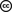Mike Beeple

# Self Organisation in Coin Selection

3 July 2018 Edsko de Vries 18 mins readThe term "self organisation" refers to the emergence of complex behaviour (typically in biological systems) from simple rules and random fluctuations. In this blog post we will see how we can take advantage of self organisation to design a simple yet effective coin selection algorithm. Coin selection is the process of selecting unspent outputs in a user's wallet to satisfy a particular payment request (for a recap of UTxO style accounting, see section "Background: UTxO-style Accounting" of my previous blog post). As Jameson Lopp points out in his blog post The Challenges of Optimizing Unspent Output Selection, coin selection is thorny problem. Moreover, there is a surprising lack of academic publications on the topic; indeed, the only scientific study of coin selection appears to be Mark Erhardt's masters thesis An Evaluation of Coin Selection Strategies.

Note: by a slight abuse of nomenclature, throughout this blog post we will refer to a user's set of unspent outputs as that user's UTxO.

## Dust

An obvious strategy that many coin selection algorithms use in some form or other is "try to get as close to the requested value as possible". The problem with such an approach is that it tends to create a lot of dust: small unspent outputs that remain unused in the user's wallet because they're not particularly useful. For example, consider the "largest first" algorithm: a simple algorithm which considers all unspent outputs of the wallet in order of size, adding them to a running total until it has covered the requested amount. Here's an animation of the effect of this algorithm: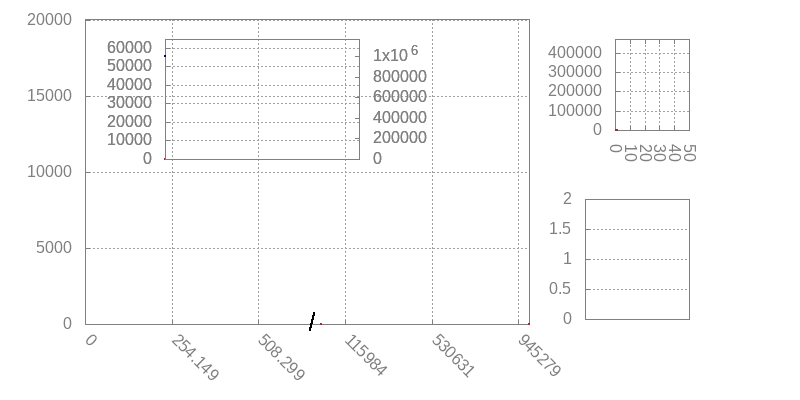Figure 1. Simulation of largest-first coin selection. Main histogram shows UTxO entries; inset graph shows UTxO balance in blue and UTxO size in red, histogram top-right shows number of inputs per transaction, graph bottom right shows the change:payment ratio (more on that below). Graph at the bottom shows the distribution of deposits (blue, left axis) versus payments (red, right axis). In this case, both are normally distributed with a mean of 1000 and 3000 respectively, and we have a deposit:payment ratio of 3:1; modelling a situation where we have frequent smaller deposits, and less frequent but larger payments (withdrawals). The wallet starts with an initial balance of 1M.

There are various things to see in this animation, but for now we want to focus on the UTxO histogram and its size. Note that as time passes, the size of the UTxO increases and increases, up to about 60k entries after about 1M cycles (with 3 deposits and 1 payment per cycle). A wallet with 60k entries is huge, and looking at the UTxO histogram we can see why this happens: virtually all of these entries are dust. We get more and more small outputs, and those small outputs are getting smaller and smaller.

## Cleaning up

Erhardt makes the following very astute observation:

If 90% of the UTxO is dust, then if we pick an unspent output randomly, we have a 90% change of picking a dust output.

He concludes that this means that a coin selection algorithm that simply picks unspent outputs at random might be pretty effective; in particular, effective at collecting dust. Indeed, it is. Consider the following simulation: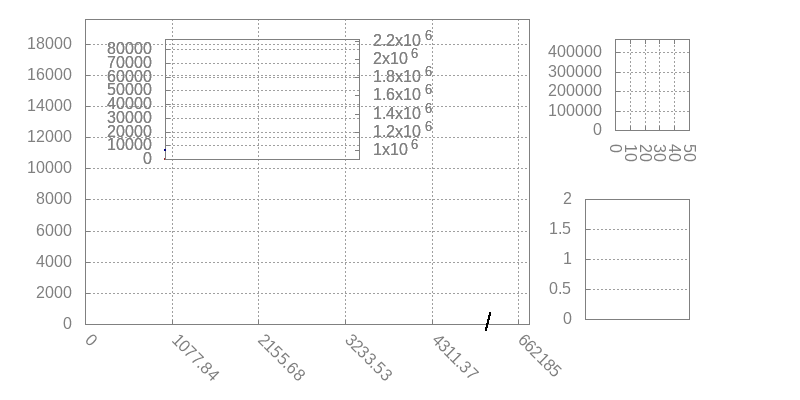Figure 2. Same distribution and ratio as in Figure 1; we run the largest-first algorithm for 1M cycles, and then random coin selection for another 150k cycles.

Notice quite how rapidly the random coin selection reduces the size of the UTxO once it kicks in. If you watch the inputs-per-transaction histogram, you can see that when the random input selection takes over, it first creates a bunch of transactions with 10 inputs (we limited transaction size to 10 for this simulation), rapidly collecting dust. Once the dust is gone, the number of inputs shrinks to about 3 or 4, which makes perfect sense given the 3:1 ratio of deposits and withdrawals.

We will restate Erhardt's observation as our first self organisation principle:

Self organisation principle 1. Random selection has a high priobability of picking dust outputs precisely when there is a lot of dust in the UTxO.

It provides a very promising starting point for an effective coin selection algorithm, but there are some improvements we can make.

## Active UTxO management

Consider the following simulation of a pure "select randomly until we reach the target value" coin selection algorithm: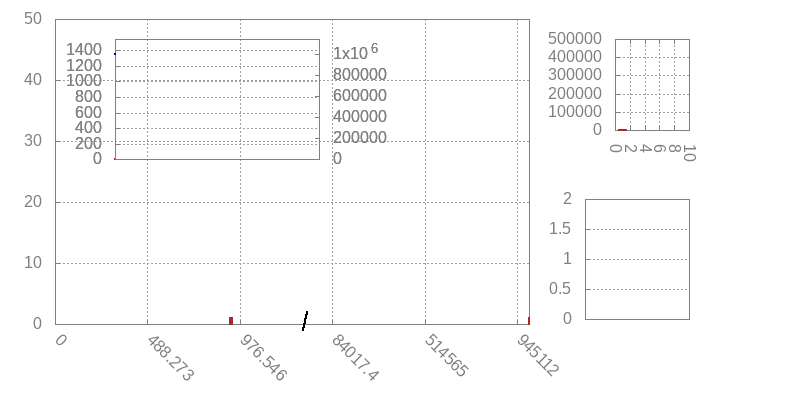Figure 3. Random-until-value-reached, for a 1:1 ratio of deposits and withdrawals, both drawn from a normal distribution with mean 1000.

The first observation is that this algorithm is doing much better than the largest-first policy in terms of the size of the UTxO, which is about 2 orders of magnitude smaller: a dramatic improvement. However, if we look at the UTxO histogram, we can see that there is room for improvement: although this algorithm is good at collecting dust, it's also still generating quite a bit of dust. The UTxO histogram has two peaks. The first one is approximately normally distributed around 1000, which are the deposits that are being made. The second one is near 0, which are all the dust outputs that are being created.

This brings us to the topic of active UTxO management. In an ideal case, coin selection algorithms should, over time, create a UTxO that has "useful" outputs; that is, outputs that allow us to process future payments with a minimum number of inputs. We can take advantage of self organisation again:

Self organisation principle 2. If for each payment request for value x we create a change output roughly of the same value x, then we will end up with a lot of change outputs in our UTxO of size x precisely when we have a lot of payment requests of size x.

We will consider some details of how to achieve this in the next section. For now see what the effect of this is on the UTxO: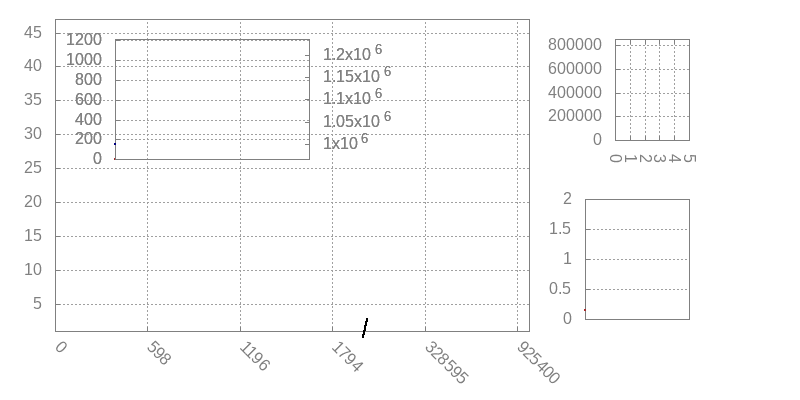Figure 4. Same deposits and withdrawals as in Figure 3, but now using the "pick randomly until we have a change output roughly equal to the payment" algorithm.

The graph at the bottom right, which we've ignored so far, records the change:payment ratio. A value near zero means a very small change output (i.e., dust); a very high value would be the result of using a huge UTxO entry for a much smaller payment. A value around 1 is perfect, and means that we are generating change outputs of equal value as the payments.

Note that the UTxO now follows precisely the distribution of payment requests, and we're not generating dust anymore. One advantage of this is that because we have no dust, the average number of inputs per transaction can be lower than in the basic algorithm.

Just to illustrate this again, here is the result of the algorithm but now with a 3:1 ratio of deposits and withdrawals: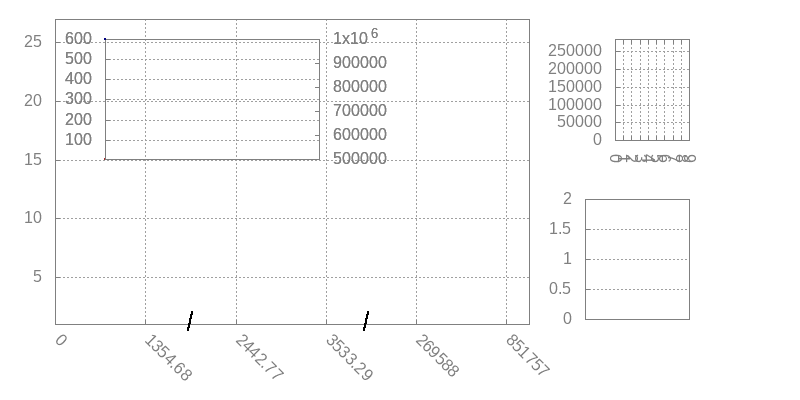Figure 5. Same algorithm as in Figure 4, but now with 3:1 deposits:payments (i.e., many small deposits, fewer but larger payments).

We have two bumps now: one normally distributed around 1000, corresponding to the the deposits, and one normally distributed around 3000, corresponding to the payment requests that are being made. As before, the median change:payment ratio is a satisfying round 1.0.

## The "Random-Improve" algorithm

We are now ready to present the coin selection algorithm we propose. The basic idea is simple: we will randomly pick UTxO entries until we have reached the required value, and then continue randomly picking UTxO entries to try and reach a total value such that the the change value is roughly equal to the payment.

This presents a dilemma though. Suppose we have already covered the minimum value required, and we're trying to improve the change output. We pick an output from the UTxO, and it turns out to be huge. What do we do? One option is to discard it and continue searching, but this would result in coin selection frequently traversing the entire UTxO, resulting in poor performance.

Fortunately, self organisation comes to the rescue again. We can set an upper bound on the size of the change output we still consider acceptable (we will set it to twice the payment value). Then we take advantage of the following property.

Self organisation principle 3. Searching the UTxO for additional entries to improve our change output is only useful if the UTxO contains entries that are sufficiently small enough. But precisely when the UTxO contains many small entries, it is less likely that a randomly chosen UTxO entry will push the total above the upper bound we set.

In other words, our answer to "what do we do when we happen to pick a huge UTxO entry?" is "we stop trying to improve our selection". We can now describe the algorithm:

1. Randomly select outputs from the UTxO until the payment value is covered. (In the rare case that this fails because the maximum number of transaction inputs has been exceeded, fall-back on the largest-first algorithm for this step.)
2. Randomly select outputs from the UTxO, considering for each output if that output is an improvement. If it is, add it to the transaction, and keep going. An output is considered an improvement when:
• It doesn't exceed the specified upper limit
• Adding the new output gets us closer to the ideal change value
• It doesn't exceed the maximum number of transaction inputs.

## Evaluation

The algorithm from Figure 6 is deceptively simple. Do the self organisation principles we isolated really mean that order will emerge from chaos? Simulations suggest, yes, it does. We already mentioned how random input selection does a great job at cleaning up dust in Figure 2; what we didn't emphasize in that section is that the algorithm we simulated there is actually our Random-Improve algorithm. Notice how the median change:payment ratio is initially very low (indicative of a coin selection algorithm that is generating a lot of dust outputs), but climbs rapidly back to 1 as soon as Random-Improve kicks in. We already observed that it does indeed do an excellent job at cleaning up the dust, quickly reducing the size of the UTxO. The simulations in Figures 4 and 5 are also the result of the Random-Improve algorithm.

That said, of course the long term effects of a coin selection algorithm can depend strongly on the nature of the distribution of deposits and payments. It is therefore important that we evaluate the algorithm against a number of different distributions.

### Normal distribution, 10:1 deposit:payment ratio

We already evaluated "Random-Improve" against normally distributed payments and deposits with a 1:1 ratio and a 3:1 ratio; perhaps more typical for exchange nodes might be even higher ratios. Here is a 10:1 ratio: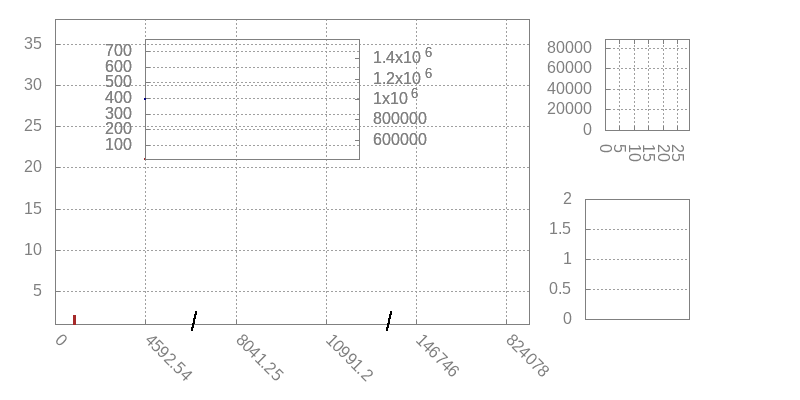Figure 7. Simulation of largest-first coin selection. Main histogram shows UTxO entries; inset graph shows UTxO balance in blue and UTxO size in red, histogram top-right shows number of inputs per transaction, graph bottom right shows the change:payment ratio (more on that below). Graph at the bottom shows the distribution of deposits (blue, left axis) versus payments (red, right axis). In this case, both are normally distributed with a mean of 1000 and 3000 respectively, and we have a deposit:payment ratio of 3:1; modelling a situation where we have frequent smaller deposits, and less frequent but larger payments (withdrawals). The wallet starts with an initial balance of 1M.

We see a very similar picture as we did in Figure 5. Since the deposits and payments are randomly drawn (from normal distributions), the UTxO balance naturally fluctuates up and down. What is satisfying to see however is that the size of the UTxO tracks the balance rather precisely; this is about as good as we can hope for. Notice also that the change:payment ratio is a nice round 1, and the average number of transaction inputs covers around 10 or 11, which is what we'd expect for a 10:1 ratio of deposits:payments.

### Exponential distribution, 1:1 deposit:payment ratio

What if the payments and deposits are not normally distributed? Here is Random-Improve on exponentially distributed inputs: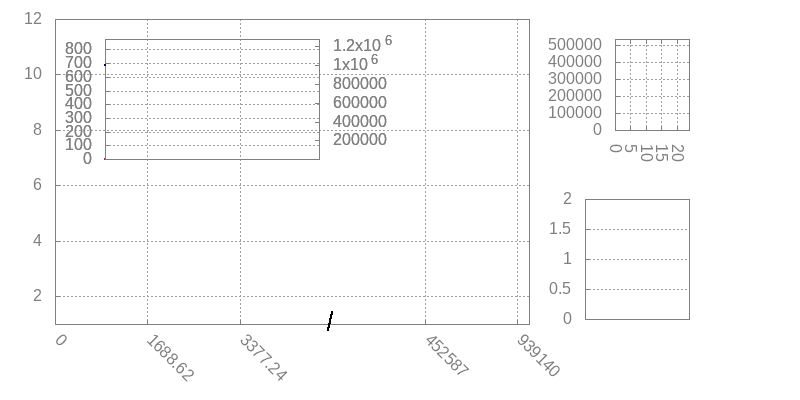Figure 8. Random-Improve, 1:1 deposit:payment ratio, deposits and payments both drawn from an exponential distribution with scale 1000.

In an exponential distribution we have a lot of values near 0; for such values it will be hard to achieve a "good" change output, as we are likely to overshoot the range. Partly due to this reason the algorithm isn't quite achieving a 1.0 change:payment ratio, but at 1.5 it is still generating useful change outputs. Furthermore, we can see that the size of the UTxO tracks the UTxO balance nicely, and the average number of transaction inputs is low, with roughly 53% having just one input.

Moreover, when we increase the deposit:payment ratio to 3:1 and then 10:0, the change:payment ratio drops to about 1.1 and then back to 1.0 (graphs omitted).

### Erlang

The exponential distribution results in many very small deposits and payments. The algorithm does better on slightly more realistic distributions such as the Erlang-k distributions (for k > 1). Here we show the animation for the 3:1 deposit:payment ratio using the Erlang-3 distribution; the results for other ratios (including 1:1) and other values of k (we additionally simulated for k = 2 and k = 10) are similar.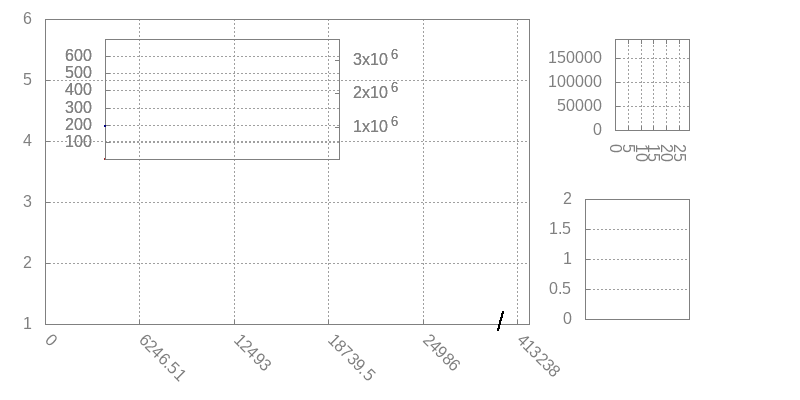Figure 9. Random-Improve, 3:1 deposit:payment ratio, deposits drawn from an Erlang-3 distribution with scale 1000 and payments drawn from Erlang-3 distributio with scale 3000.

### More payments than deposits

We have been focusing on the case where we have more deposits and fewer (but larger) payments. What happens if the ratio is reversed?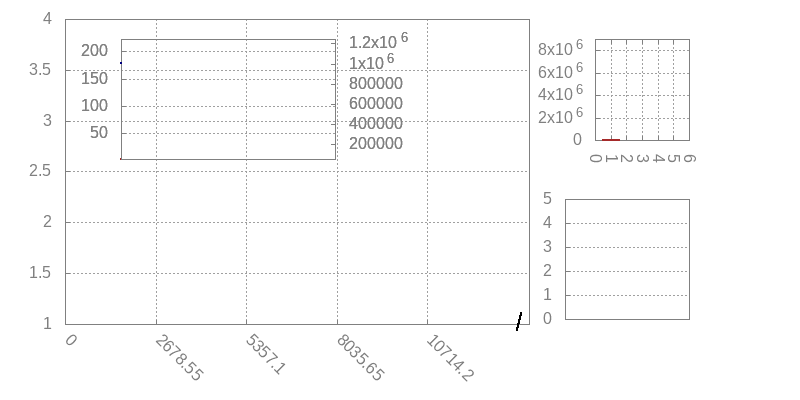Figure 10. Random-Improve, 1:10 deposit:payment ratio, deposits and payments drawn from a normal distribution with mean 10k and 1k, respectively. 1M cycles.

In this case we are unable to achieve that perfect 1.0 change:payment ratio, but this is expected: when we have large deposits, then we frequently have no choice but to use those, leading to large change outputs. We can see this more clearly when we slow things right down, and remove any source of randomness; here is the same 1:10 ratio again, but now only the first 100 cycles, and all deposits exactly 10k and all payments exactly 1k: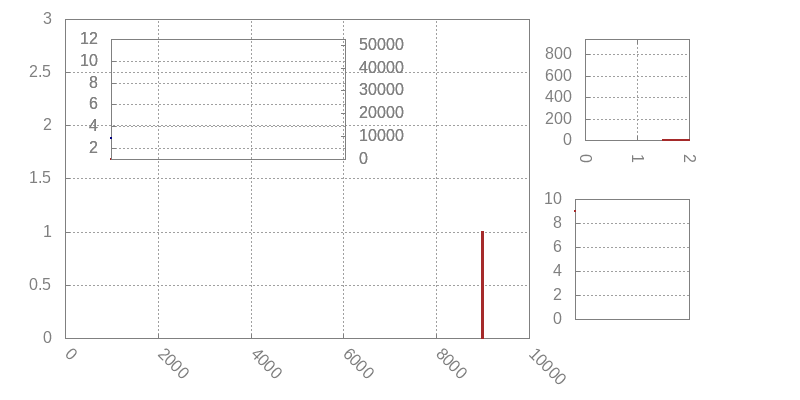Figure 11. Random-Improve, 1:10 deposit:payment ratio, all deposits exactly 10k, all payments exactly 1k (no randomness). First 100 cycles only.

We can see the large value being deposited, and then shifting to the left in the histogram as it is getting used for deposits, each time decreasing that large output by 1k. Indeed, this takes 10 slots on average, which makes sense given the 10:1 ratio; moreover, the average value of the "large output" in such a 10-slot cycle is 5k, explaining why we are getting 5.0 change:payment ratio.

The algorithm however is not creating dust outputs; the 1k change outputs it is generating are getting used, and the size of the UTxO is perfectly stable. Indeed, back in Figure 12 we can see that the size of the UTxO tracks the balance perfectly; moreover, the vast majority of transactions only use a single input, which is what we'd expect for a 10:0 deposit:payment ratio.

### Real data

#### MoneyPot.com

Ideally, of course, we run the simulation against real event streams from existing wallets. Unfortunately, such data is hard to come by. Erhardt was able to find one such dataset, provided by MoneyPot.com. When we run our algorithm on this dataset we get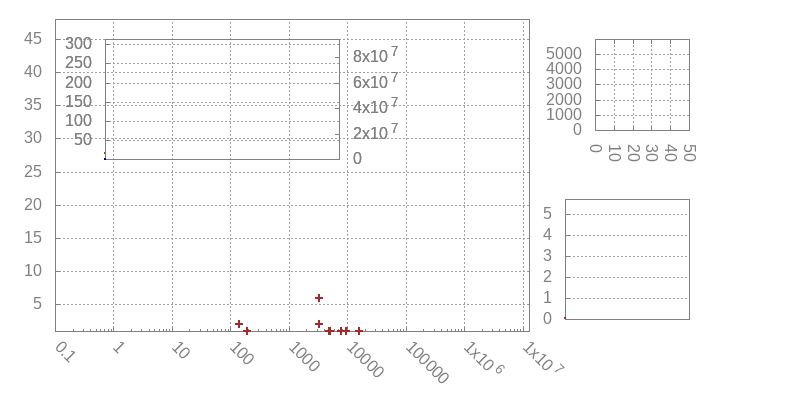Figure 12. Random-Improve, using the MoneyPot data set. There is a rougly 2:1 deposit:payment ratio. Values have been scaled. NOTE: log scale on the x-axis.

A few observations are in order here. First, there are quite a few deposits and payments close to 0, just like in an exponential distribution. Moreover, although we have many values close to 0, we also have some huge outliers; the payments are closely clustered together, but there is a 10xE9 difference between the smallest and largest deposit, and moreover a 10xE5 difference between the largest deposit and the largest payment. It is therefore not surprising that we end up with a relatively large change:payment ratio. Nonetheless, the algorithm is behaving well, with the size of the UTxO tracking the balance nicely, with an average UTxO size of 130 entries. The average number of outputs is 3.03, with 50% of transactions using just one input, and 90% using 6 or fewer.

#### Cardano Exchange

One of the large Cardano exchange nodes has also helped us with some anonymised data (deposits and payments), similar in nature to the MoneyPot dataset albeit significantly larger. Coming from an exchange node, however, this dataset is very much skewed towards deposits, with a deposit:payment ratio of roughly 30:1. Under these circumstances, of course, coin selection alone cannot keep the UTxO size small.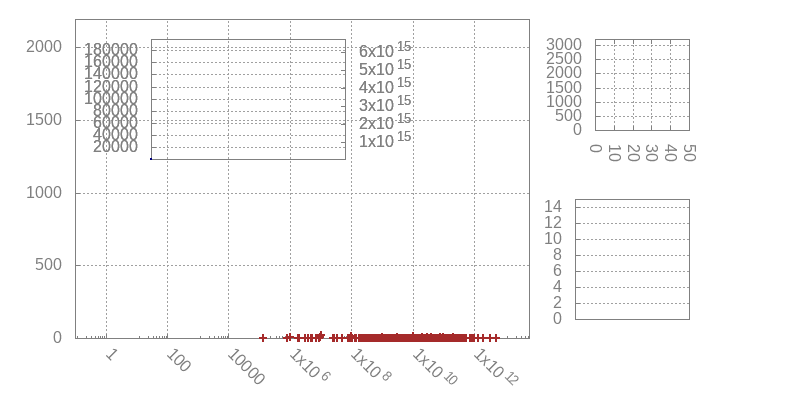Figure 13. Random-Improve, using data set from a large Cardano exchange. There is a rougly 30:1 deposit:payment ratio. Values have been scaled. NOTE: log scale on the x-axis.

## Conclusions

The choice of coin selection algorithm has far reaching consequences on the long term behaviour of a cryptocurrency wallet. To a large extent the coin selection algorithm determines, over time, the shape of the UTxO. Moreover, the performance of the algorithm can be of crucial importance to high-traffic wallets such as exchange nodes.

In his thesis, Erhardt proposes "Branch and Bound" as his preferred coin selection algorithm. Branch and Bound in essence is a limited backtracking algorithm that tries to find an exact match, so that no change output needs to be generated at all. When the backtracking cannot find an exact match within its bounds, the algorithm then falls back on random selection. It does not, however, attempt our "improvement" step, and instead just attempts to reach a minimum but fixed change size, to avoid generating dust. It is hard to compare the two algorithms directly, but on the MoneyPot dataset at least the results are comparable; Erhardt ends up with a slightly smaller average UTxO (109 versus our 130), and a slightly smaller average number of inputs (2.7 versus our 3.0). In principle we could modify our Random-Improve algorithm to start with bounded backtracking to find an exact match, just like Erhardt does; we have not done this however because it adds complexity to the algorithm and reduces performance. Erhardt reports that his algorithm is able to find exact matches in 30% of the time. This is very high, and at least partly explains why his UTxO and average number of change outputs is lower; in the Cardano blockchain, we would not expect that there exist exact matches anywhere near that often (never mind finding them).

Instead our proposed Random-Improve does no search at all, instead purely relying on self organisation principles, the first of which was stated by Erhardt, and the other two we identified as part of this research. Although in the absence of more real data it is hard to evaluate any coin selection algorithm, we have shown that the algorithm performs well across a large variety of different distributions and deposit:payment ratios. Moreover it is straight-forward to implement and has high performance.

One improvement we may wish to consider is that when there are very large deposits, we could occassionally issue a "reorganisation transaction" that splits those large deposits into smaller chunks. This would bring the change:payment ratio down, which would improve the evolution of the UTxO over time and is beneficial also for other, more technical reasons (it reduces the need for what we call "dependent transactions" in the wallet specification). Such reorganisation is largely orthogonal to this algorithm, however, and can be implemented independently.

# Semi-Formal Development: The Cardano Wallet

4 June 2018 Edsko de Vries 17 mins readPlease note: this post originally appeared on the Well-Typed blog.

TL;DR: A combination of formal modelling and testing using QuickCheck is a powerful tool in the design and implementation of high assurance software. Consistency of the model can be checked by testing invariants, and the real implementation can be tested by comparing it against the model.

As part of our consulting work for IOHK, Well-Typed have been working with IOHK on the design and implementation of the new version of the Cardano cryptocurrency wallet. As a crucial component of this process, we have written a semi-formal specification of the wallet: a mathematical model of the wallet along with invariants and lemmas about how it behaves.

We refer to this specification as “semi-formal” because while it states many of the wallet’s properties, and proves some of them, it by no means proves all of them. As we will see, however, we can use QuickCheck to test such properties, producing counter-examples where they fail to hold. Not only is this an invaluable tool during the development of the specification itself, it also gives us a very principled way of testing the real implementation, even if later we do prove all the remaining properties as well.

In this blog post we will take a look at the specification and see how it drives the design and testing of the new wallet. We will show parts of the formal development, but only to give some idea about what it looks like; we will not really discuss any of its details. The goal of this blog post is not to describe the mathematics but rather the approach and its advantages.

Note: all figure, invariant and section numbers used in this blog post refer to version 1.1 of the specification.

## Background: UTxO-style Accounting

Regular bank transactions transfer from and to bank accounts. For example, a transaction might transfer \$100 from Alice to Bob. Transactions in cryptocurrencies such as Cardano or Bitcoin are a little different. The to part is similar, except that there might be multiple “outputs”; for example, a transaction might transfer \$70 to Bob and \$30 to Carol. The from part however works in quite a distinct way; transaction inputs are not accounts but other transactions. For example, let’s call the transaction that transfers \$70 to Bob and \$30 to Carol “t1”.

``````t1 	inputs:	…
outputs:	\$70 to Bob, \$30 to Carol``````

Now suppose Bob wants to transfer \$50 to Dave. He can create a new transaction that says “take the first output of transaction t1, transfer \$50 to Dave and transfer \$20 back to me”1.

``````t2	inputs:	first output of t1
outputs:	\$50 to Dave, \$20 to Bob``````

It is important that Bob transfers the \$20 “change” back to himself, because a transaction output can be “spent” (that is, used as an input) only once. This style of transactions is called “UTxO” style; UTxO stands for “Unspent Transaction (Tx) Outputs”.

The blockchain is basically a long list of such transactions. The corresponding formal definition looks something like this.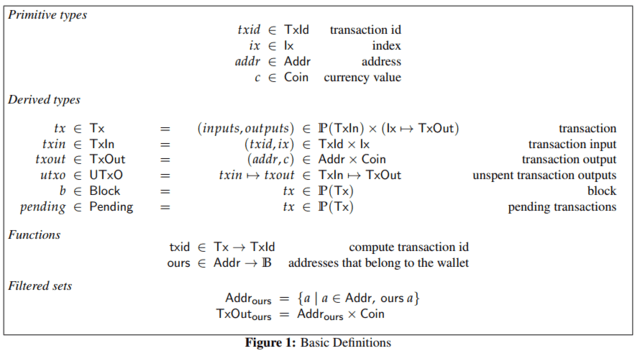## Wallet

The cryptocurrency’s wallet is a piece of software that monitors the state of the blockchain, keeps track of the user’s funds (more precisely, their UTxO) and enables them to create new transactions to be included into the blockchain. The wallet is the primary means by which users interact with the blockchain. Note that the verification of these new transactions and the decision whether or not to include them into the global blockchain is not up to the wallet; how this happens is beyond the scope of this blog post.

A formal specification of the wallet is a mathematical abstraction that strips away all irrelevant detail and just focuses on the core functionality of the wallet. In the basic model of the Cardano wallet, we stripped down the wallet state to just the wallet’s UTxO and its set of pending transactions; the specification is small enough to fit on a single page.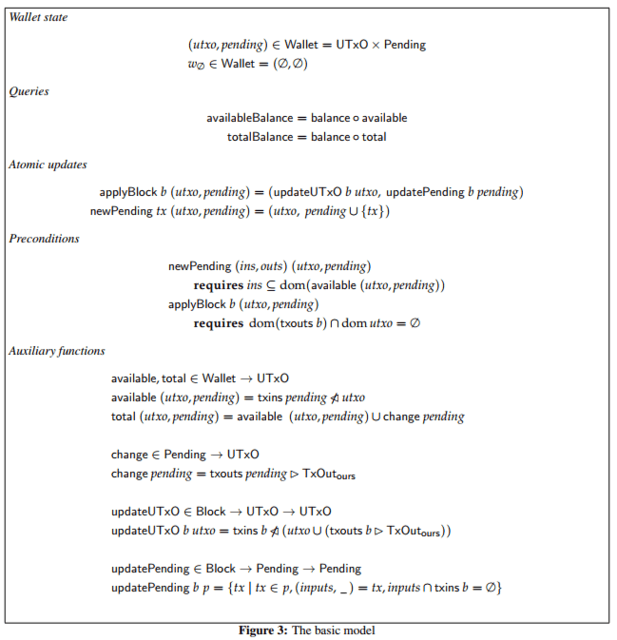Such a model is simple enough to be studied in-depth and support mathematical proofs of its properties. Yet it is accurate enough to be an invaluable guide in the design of the wallet and be the base for unit tests that drive the real implementation. It can also be used to study where we most need to worry about performance and how we can address those concerns.

## Balance

As an example of one of the trickier aspects of the wallet’s design, we will discuss reporting balance in a bit more detail. It may be surprising that reporting balance is non-trivial, but even for regular bank accounts we already have two notions of balance. After Alice transfers \$100 to Bob, her online bank account might tell her

• Your current balance is \$1000.
• There is a pending transaction of \$100, so your available balance is \$900.

Unlike regular bank transactions, UTxO-style transactions involve change; this leads naturally to three notions of balance. If we take as example transaction t2 above, Bob’s balance might be reported as

• Your UTxO balance is \$1070.
• There is a pending transaction t2, so your available balance is \$1000.
• However, transaction t2 returns \$20 in change, so your total balance is \$1020.

Note that the change from t2 becomes available only when transaction t2 has been included into the blockchain.

Although there are user interface questions here (how should we report these different kinds of balance to the user?), from the wallet design perspective this is not yet particularly difficult. The real complexity comes from the fact that there may be temporary disagreement about which transactions are included in the blockchain (such disagreements are known as “forks”; how they arise and are resolved is again well beyond the scope of this blog post).

Let’s stick to the above example, and suppose that t2 is pending, but there was disagreement about t1. After the disagreement got resolved the wallet discovers that t1 is not actually present in the blockchain after all (perhaps it will be later). The wallet’s available balance is now still \$1000, but would it really make sense to report its total balance as \$1020? It would be strange to have the total balance be larger than the UTxO balance; not wrong per se, but confusing nonetheless.

In the specification we therefore define the concept of minimum balance: the minimum (UTxO) balance of the user “across all possible futures”; this is the only balance the user can be certain of. In the example, this is the future in which neither t1 nor t2 ever make it into the blockchain, and hence we report \$1000 as the minimum balance (note that it can never happen that t2 is included but t1 is not, since t2 depends on t1). While this concept makes intuitive sense, in order to make it precise and be able to compute it we needed to introduce the concept of “expected UTxO”: unspent transaction outputs that the wallet expects will become available in the future but haven’t yet.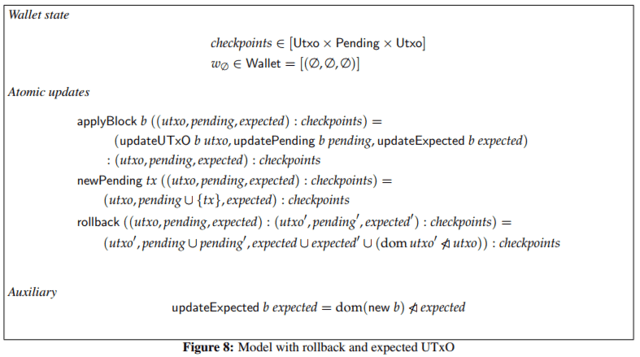Of course, even without a formal specification it is possible that we could have come up with this concept of minimum balance and a way to compute it. But having the formal model allows us to think much more clearly about the problems, remove all the clutter that surrounds a “real” implementation of the wallet and focus only on the core ideas.

## Internal consistency: invariants

When we introduce a novel concept such as “expected UTxO” into the specification, there is a tricky question: how do we know that what we specified is right? Actually, since we introduced the concept ourselves, the question of whether it was “right” or not is not particularly meaningful. Instead we ask: is what we specified useful?

One way to answer this question is by stating invariants. Invariants are properties that we expect to be true at all times. For example, for the basic model shown above (Figure 3) we have the following invariant:

``Invariant 3.4. txins pending ⊆ dom utxo``

What this invariant says is that the pending transactions can only spend from the wallet’s unspent outputs. Intuitively, this makes a lot of sense: the wallet should not allow the user to spend funds they don’t have. As we have seen however in the previous section, this invariant does not always hold when we take disagreements about the blockchain into account! When Bob submits transaction t2, spending an output of transaction t1, and only later discovers that actually t1 should not have been included in the blockchain after all, he will have spent an output that is not in his UTxO.

The concept of expected UTxO comes to the rescue, again. The invariant for the full model is instead:

``Invariant 7.8. txins pending ⊆ dom (utxo ∪ expected)``

In other words, pending transactions can only spend available outputs or outputs that we expect to become available (because they were previously).

Another useful invariant that helps further solidify our intuition about what we mean by expected UTxO says that an output can never be in both the actual UTxO and the expected UTxO

``Invariant 7.6. dom utxo ∩ dom expected = ∅``

After all, it would be strange to say that an output is expected if we already have it in our wallet. Stating invariants like this allows us to make our intuitions about the concepts we introduce precise, and proving them gives us strong guarantees that our specification makes sense and is internally consistent.

## Semi-formal development

Formally proving invariants such as the ones discussed above can be time consuming and requires mathematical training. Naturally, doing the proofs would be ideal, but the main point of this blog post is that we push this approach a long way even if we don’t. After all, we program in Haskell precisely because we can easily translate back and forth between Haskell and mathematics.

To translate the various wallet models from the specification, we use the approach we described in a previous blog on Object Oriented Programming in Haskell (indeed, we developed that approach specifically for this purpose). For instance, here is the Haskell translation of the basic model:

``````mkWallet :: (Hash h a, Ord a, Buildable st)
=> Ours a -> Lens' st (State h a) -> WalletConstr h a st
mkWallet ours l self st =
(mkDefaultWallet (l . statePending) self st) {
utxo       = st ^. l . stateUtxo
, ours       = ours
, applyBlock = \b -> self (st & l %~ applyBlock' ours b)
}

applyBlock' :: Hash h a
=> Ours a -> Block h a -> State h a -> State h a
applyBlock' ours b State{..} = State {
_stateUtxo    = updateUtxo ours b _stateUtxo
, _statePending = updatePending   b _statePending
}

updateUtxo :: forall h a. Hash h a
=> Ours a -> Block h a -> Utxo h a -> Utxo h a
updateUtxo p b = remSpent . addNew
where
addNew, remSpent :: Utxo h a -> Utxo h a
addNew   = utxoUnion (utxoRestrictToOurs p (txOuts b))
remSpent = utxoRemoveInputs (txIns b)

updatePending :: Hash h a
=> Block h a -> Pending h a -> Pending h a
updatePending b = Map.filter \$ \t -> disjoint (trIns t) (txIns b)``````

It deals with more details than the specification; for instance, it explicitly abstracts away from a specific choice of hash h, as well the types of addresses a. It is therefore a bit more complicated than the spec, but it nonetheless follows the specification very closely. In particular, it is still a model: it does not deal with any networking issues or persistent storage, may not be particularly performant, etc. In other words, this is not intended to be the design of the real wallet. Having this model is nonetheless incredibly useful, for two reasons. The first is that we can use it to test the real wallet; we will discuss that in the next section.

The second reason is that we can use the model to test the invariants. For example, here is the translation of Invariants 7.8 and 7.6 from the previous section to Haskell:

``````pendingInUtxoOrExpected :: WalletInv h a
pendingInUtxoOrExpected l e =
invariant (l <> "/pendingInUtxoOrExpected") e \$ \w ->
checkSubsetOf
("txins pending",
txIns (pending w))
("utxo ∪ expected",
utxoDomain (utxo w) `Set.union` utxoDomain (expectedUtxo w))

utxoExpectedDisjoint :: WalletInv h a
utxoExpectedDisjoint l e =
invariant (l <> "/utxoExpectedDisjoint") e \$ \w ->
checkDisjoint
("dom utxo",
utxoDomain (utxo w))
("dom expected",
utxoDomain (expectedUtxo w))``````

As for the wallet implementation, the Haskell translation of the invariants deals with more details than the spec; in this case, one of the main differences is that the infrastructure for these invariants is designed to give detailed error reports when the invariants do not hold. Nonetheless, the main part of the invariant is again a direct translation of the specification.

The big gain is that we can now use QuickCheck to test these invariants. We generate random (but valid) events for the wallet (“apply this block”, “submit this new transaction”, “switch to a different fork”) and then check that the invariants hold at each point. For example, in the first release of the wallet specification there was a silly mistake: when the wallet was notified of a new block, it removed the inputs of that block from the expected UTxO, rather than the outputs. A silly mistake, but easy to miss when just reviewing the specification by hand. A proof would have found the mistake, of course, but so can QuickCheck:

``````Wallet unit tests
Test pure wallets
Using Cardano model FAILED 

Failures:

test/unit/Test/Spec/Models.hs:36:
1) Wallet unit tests, Test pure wallets, Using Cardano model
predicate failed on: Invalid [] InvariantViolation {
name:     full/utxoExpectedDisjoint
, evidence: NotSubsetOf {
dom utxo: ..
, dom expected: ..
, dom utxo `intersection` dom expected: ..
}
, events:   {
state: ..
, action: ApplyBlock ..
, state: ..
, action: NewPending Transaction{ .. }
, state: ..
..
, action: Rollback
..
}
}``````

Not only does this tell us that the invariant did not hold; it actually gives us the specific sequence of events that lead to the wallet state in which the invariant does not hold (as well as all the intermediate wallet states), and it tells what the domain of the UTxO and the expected UTxOs are in that state as well as their intersection (which should have been empty but wasn’t).

## Testing the real implementation

As mentioned, the Haskell translation of the wallet specification is still a model which ignores a lot of the real world complexity that the full wallet implementation must deal with. Even the datatypes that the model works with are simplified versions of the real thing: transactions don’t include signatures, blocks are not real blocks but just lists of transactions, etc.

Nonetheless, we can use the model to test the real implementation also. We can translate the simplified types of the model to their real counterparts. Since we have QuickCheck generators for the simplified types and we can test the model because we have an implementation of it, we can test the real wallet as shown in the following commuting diagram: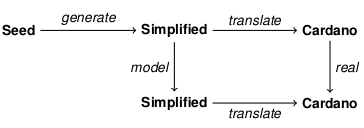In words, what this means is that we use the QuickCheck generator to generate wallet events using the simplified model types and then do two things:

• We execute the model on the simplified types and translate after.
• We translate first and then execute the real wallet on the translated input.

Either way, we end up with two final answers (both in terms of the real Cardano types), one executed in the model and one executed in the real wallet. We can compare these two, and if they match we can conclude that the real wallet implements the model correctly. Since we check this property at each step and we know that the invariants hold in the model at each step, we can then also conclude that the invariants hold in the real implementation at each step.

For example, when a bug in the full wallet meant that change from pending transactions was sometimes double-counted (in the case where pending transactions use the change of other pending transactions as inputs, a case that can only happen in the presence of forks), the generator will be able to find a counter-example to the above commuting diagram, and then give us the exact sequence of wallet events that leads to a wallet state in which the real wallet and the model disagree as well as the precise value that they disagree on.

## Conclusions

Software specifications, where they exist at all, are often informal documents that describe the features that the software is intended to have in natural language. Such specifications do not lend themselves easily to verification or even testing. At the other end of the spectrum, fully formal specifications where every property is verified mathematically are costly and time consuming to produce and require specialized expertise. They are of course the golden standard, but there is also a useful middle-ground: by specifying the model and its properties formally, we can test its properties using QuickCheck. Moreover, we get a model in which we can reason about the core functionality of the application and which we can then compare against the real implementation.

IOHK’s development of the new wallet is open source and can be found on GitHub. Excitingly, IOHK have recently hired someone to start work on the Coq formalization of the wallet specification, which will put the whole specification on an even stronger footing. Of course, that does not make any of the existing work useless: although it will become less important to test the invariants in the model, having the QuickCheck generators available to check the real implementation is still very valuable. Moreover, having the QuickCheck tests validate the invariants before we attempt to prove them formally can also save valuable time if we discover early that an invariant is not, in fact, true.

## References

1. We ignore transaction fees for the sake of simplicity.

Artwork,Mike Beeple

# Writing a High-Assurance Blockchain Implementation

### Guest blog from Edsko de Vries, who is working on the high assurance implementation of the Ouroboros blockchain protocol

3 November 2017 Edsko de Vries 9 mins read

Writing a High-Assurance Blockchain Implementation - Input OutputIn our previous blog post Cryptocurrencies need a safeguard to prevent another DAO disaster we discussed the need for high assurance development of cryptocurrencies and their underlying blockchain protocols. We also sketched one way in which one might go about this, but we did not give much detail. In this follow-up blog post we delve into computer science theory a bit more, and in particular we will take a closer look at process algebras. We will have no choice but omit a lot of detail still, but hopefully we can develop some intuition and provide a starting point for reading more.

## Compositionality

When we want to study computer programs as mathematical objects that we can manipulate and analyze, we need to write them in a programming language for which we have a well understood mathematical theory. If this mathematical theory inherits much of what we know from elementary mathematics, that's an additional benefit. For example, we learn in school that:

``for every number n, n + n = 2 * n``

Shockingly, in many programming languages even this most basic of equations does not hold. For example, in the language C, when we define

``````int f() {
return 5;
}``````
``````int g() {
int x;
scanf("%d", &x);
return x;
}``````

we have that while f() + f() is the same as 2 f() (both equate to 10), g() + g() is certainly not the same as 2 g(): the former asks the user for two numbers and adds them together, the latter asks the user for a single number and multiplies it by two. In the language Haskell this distinction between an honest-to-goodness number, and a program that returns a number is explicit (this is what we mean when we say that Haskell is a pure language), and for numbers basic mathematical equations such as the above hold true. Knowing that for every number n, n + n = 2 n in Haskell would still not be particularly useful without another property of Haskell: when we see the expression n + n anywhere in a program, we can (almost) always replace it with 2 n and know that our program still behaves in the same way: we say that Haskell is referentially transparent. This is key: it means that we can analyze bits of our program at a time and know that our conclusions are also correct in the wider context of the whole program. In other words, it means that we can do compositional reasoning: we can understand the larger program by understanding its components individually. This is essential when dealing with anything but toy examples.

## Process Algebra

Of course, even in Haskell we can read from files, write to network sockets, etc. and while the type system makes sure that such programs are distinguished from pure values, reasoning about such programs is nonetheless more difficult because we do not have a good mathematical theory that covers all of these "effects". It is therefore useful to identify a subset of effects for which we do have a mathematical theory. Different kinds of applications need different subsets of effects. For some applications a process algebra is a good match. A process algebra is a programming language in which we can express systems of concurrent programs that communicate with each other, along with a mathematical theory. There are many different kinds of process algebras, and they differ in the details. For example, some provide synchronous point to point communication, others asynchronous; a few provide broadcast communication. So what would be an analogue to a law like n + n = 2 * n in such a language? Well, there are multiple answers, but one answer is bisimilarity, which intuitively allows us to say that "two processes behave the same". For example, consider the two following processes

``````p1 m = forever \$ do
bcast m
bcast m``````
``````p2 m = forever \$ do
bcast m``````

Both p1 and p2 consist of an infinite loop; p1 broadcasts message m twice within that loop, and p2 only once. While these two processes may look different, they really aren't: both broadcast message m indefinitely. To make "they aren't really different" more precise, we can try to prove that p1 and p2 are bisimilar. Intuitively, this means that we have to show that any action that p1 can take, p2 can also take, and they are still bisimilar after taking those actions. The proof for p1 and p2 would look something like this, where a red line indicates "can do the same action here":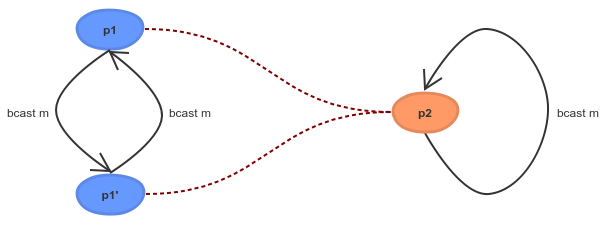So why is this useful? When two processes are bisimilar, you know three things: they can do the same actions, they will continue to be able to do the same actions, and most importantly, this is compositional: if p1 is bisimilar to p2 then we can replace p1 with p2 in (most) contexts. As before, this is essential: it means we can understand and analyze a larger program by understanding and analyzing its components.

## Psi-calculus

Process algebra is a large field in computer science, and many different kinds of algebras have been studied and are being studied. Some of the earliest and perhaps most well-known are the Calculus of Communicating Systems (CCS) and Communicating Sequential Processes (CSP). These days one of the most important process algebras is the pi calculus; argueably, the pi calculus is to concurrent programming what the lambda calculus is to functional programming. While the lambda calculus forms the theoretical basis for functional programming, nobody actually writes any code in the lambda calculus; it would be akin to writing in assembly language. Instead we write programs in a much more sophisticated functional language like Haskell. Similarly, the pi calculus is a "core" calculus, good for foundational research but not so good for actually writing programs. Instead, we will use the psi calculus which is a generalization of the pi calculus with more advanced features, but still with a well understood theory. Let's consider an example of bisimilarity in the psi calculus, similar to the previous example but slightly more interesting. Do you think we should regard processes p3 and p4 as "behaving the same"?

``````p3 m = forever \$ do
k  <- newKey ; bcast (encrypt k  m)
k' <- newKey ; bcast (encrypt k' m)

p4 m = forever \$ do
k  <- newKey ; bcast (encrypt k m)``````

What about process p5 and p3?

``````p5 m = forever \$ do
k <- newKey
bcast (encrypt k m)
bcast (encrypt k m)``````

It turns out process p3 and p4 are bisimilar, and the proof is almost the same as before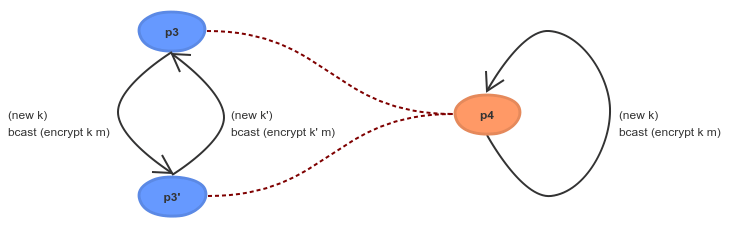They both send out an infinite number of messages, each message encrypted with a new key. Although p3 and p4 will generate different keys, from the outside we cannot tell them apart because we don't know anything about those keys. Process p5 and p4 are however not bisimilar: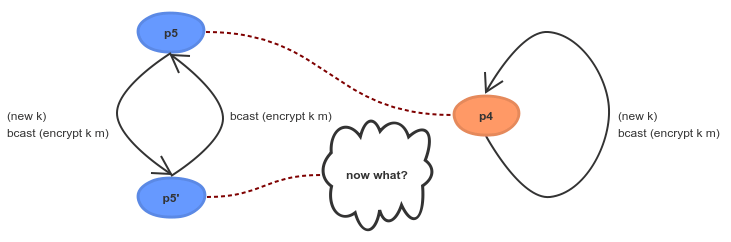After p5 sends out a message encrypted with some key k, it then sends that message again encrypted with the same key; p4 cannot follow.

## Ouroboros Praos

One of the nice things about the psi calculus is that we can embed it as a domain-specific language (DSL) in Haskell. We use this to define a model of the Ouroboros Praos blockchain protocol, which we can both execute and run (it's just Haskell after all), and even test using QuickCheck (Haskell's randomized testing tool), but moreover is also amenable to formal verification using the psi calculus metatheory.

We start by expressing the algorithm as it is defined in the Ouroboros Praos paper in our Haskell psi calculus embedding. Although we cannot formally verify that this algorithm matches the one in the paper, the correspondence is close enough that it can easily be verified by hand. We can run this implementation and experiment with it. This first implementation will do various things that, while we can implement them, we would not want to do in a real implementation. So we then make a number of small, incremental adjustments, that get us closer and closer to an actual high performance implementation. Each of the individual adjustments should be small enough that a human reader can understand how we go from one implementation to the next and convince themselves that we haven't changed anything fundamental.

Each of these algorithms can be run, so that we can test that all of the adjustments we make to the original algorithm don't change any fundamental properties. Since we express the algorithm in terms of a well-understood process calculus, we can also take some of these adjustments and formally prove that we haven't changed anything fundamental. Bisimulation is but one tool in the theoretical toolbox here; there are many others. The end result is that we have a bridge from the high level specification of the algorithm in the academic literature to the actual low level implementation that we implement, with high assurance that what we actually run indeed corresponds to the algorithm defined and proved correct by the cryptographers.